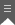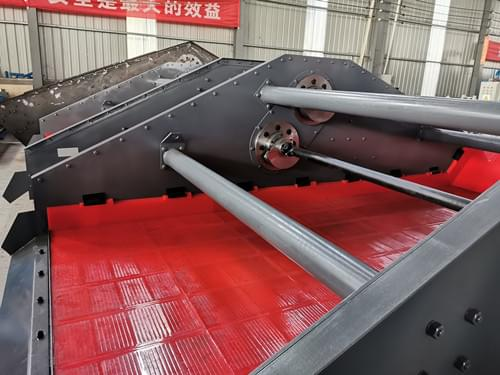﻿ How Do You Calculate Dewatering Volume?
Our LocationLocation: Home » SOLUTIONS » PROJECT CASE » How Do You Calculate Dewatering Volume?

# How Do You Calculate Dewatering Volume?

Dewatering volume is the amount of water that needs to be removed from a material before it can be used or processed. There are several methods for calculating dewatering volume, depending on the specific application and the type of material being dewatered. Here are some common methods:Gravity method: This involves measuring the weight of a sample of the material being dewatered and then removing the water until the weight of the material is equal to the original weight. The amount of water removed can then be calculated as a percentage of the original weight.

Mass balance method: This involves measuring the mass of a sample of the material being dewatered and then removing the water until the mass of the material is equal to a certain threshold value. The amount of water removed can then be calculated as a percentage of the original mass.

Volumetric method: This involves measuring the volume of a sample of the material being dewatered and then removing the water until the volume of the material is equal to a certain threshold value. The amount of water removed can then be calculated as a percentage of the original volume.

Overall, there are many different methods for calculating dewatering volume, and the specific method used will depend on the application and the type of material being dewatered. It’s important to choose an appropriate method to ensure accurate results.

### Send Message

Please write down your requirement and contact details in the following form. You can also send a message to us by this email export@lylzzg.com, we will reply to you within 24 hours.